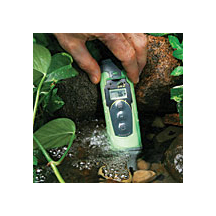# pH MeasurementpH measurement is used in a wide variety of applications: agriculture, wastewater treatment, industrial processes, environmental monitoring, and in research and development. pH is a measure of the acidity or alkalinity of a solution. The pH value states the relative quantity of hydrogen ions (H+) contained in a solution. The greater the concentration of H+ the more acidic the solution and the lower the pH. In this relationship, pH is defined as the negative logarithm of hydrogen activity. A standard pH measuring system consists of three elements: 1) pH electrode; 2) temperature compensation element and 3) pH meter or controller.

Definition of pH
The pH value of a solution is the negative log of its hydrogen ion activity (α), which is the product of hydrogen ion concentration [H+] and the activity coefficient of hydrogen (gH+) at that concentration.

pH = –log α = –log γH+[H+]

In pure water and in dilute solutions, the H+ activity can be considered the same as the H+ concentration.

pH = –log γH+[H+] = –log [H+]

The pH of a solution measures the degree of acidity or alkalinity relative to the ionization of water. Pure water dissociates to yield 10-7 M of [H+] and [OH–] at 25°C; thus, the pH of water is 7, the point of neutrality.

pHwater = –log [H+] = –log 10-7 = 7

Most pH readings range from 0 to 14. Solutions with a higher [H+] than water (pH less than 7) are acidic; solutions with a lower [H+] than water (pH greater than 7) are basic or alkaline.Definition of pH
Measuring pH involves comparing the potential of solutions with unknown [H+] to a known reference potential. pH meters convert the voltage ratio between a reference halfcell and a sensing half-cell to pH values. Today, most electrodes are combination electrodes with both the reference and sensing half-cells in the same body.

Reference Half-Cells contains a conductor (usually silver with a silver chloride coating) immersed in a solution with known [H+]. The potential between this internal conductor and the known solution is constant, providing a stable reference potential.

Sensing Half-Cells (measuring half-cells) are made of a nonconducting glass (or epoxy) tube sealed to a conductive glass membrane. Like the reference half-cell, the sensing half-cell also contains a conductor immersed in a buffered electrolyte solution, ensuring constant voltages on the inner surface of the glass membrane and the sensing conductor.

When the pH electrode is immersed in the solution to be measured, a potential is established on the surface of the sensing glass membrane. If the unknown solution  is neutral, the sum of fixed voltages on the inner surface of the glass membrane and on the sensing conductor approximately balances the voltage on the outer surface of the glass membrane and the reference half-cell. This results in a total potential difference of 0 mV and a pH value of 7.

In acidic or alkaline solutions, the voltage on the outer membrane surface changes proportionally to changes in [H+]. The pH meter detects the change in potential and determines [H+] of the unknown by the Nernst equation:where:

• E = total potential difference (measured in mV)
• T = temperature in Kelvin
• n = number of electrons
• E° = reference potential
• F = Faraday’s constant
• R = gas constant
• [H+] = hydrogen ion concentration

pH Temperature Compensation
The pH of any solution is a function of its temperature.  Voltage output from the electrode changes linearly in relationship to changes in pH, and the temperature of the solution determines the slope of the graph. One pH unit corresponds to 59.16 mV at 25°C, the standard voltage and temperature to which all calibrations are referenced. The electrode voltage decreases to 54.20 mV/pH unit at 0.0°C and increases to 74.04 mV/pH unit at 100.0°C. (See graph below.)typical pH electrode response as a function of temperature

Since pH values are temperature dependent, pH applications require some form of temperature compensation to ensure standardizes pH values.  Meters and controllers with automatic temperature compensation (ATC) receive a continuous signal from a temperature element and automatically correct the pH value based on the temperature of the solution.  Manual temperature compensation requires the user to enter the temperature. ATC is considered to be more practical for most pH applications.